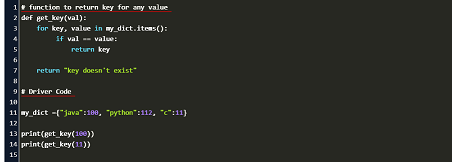# How to Get Key from Value in Python Dictionary

Python dictionary allows you to store different data types in a compact structure. Sometimes you may need to get key from value in Python dictionary, sort of a reverse lookup in Python dictionary. There are a couple of easy ways to do this in Python. In this article, we will look at those methods to lookup value in dictionary and retrieve key.

## How to Get Key from Value in Python Dictionary

Here are a couple of ways to get key from value in python dictionary.

## 1. Using list.index

In this approach, we first create two lists – one for all the keys and the other for all the values in our dictionary. Then we get the index position of value in the second list using list.index() function. Once we get the index position, we use it to retrieve key value from the first list. Here is an example.

````# creating a new dictionary`
`dict1 ={"a":1, "b":2, "c":3}`

`# list out keys and values separately`
`key_list =` `dict1.keys()`
`val_list =` `dict1.values()`

`# get position of val`ue 2 in second list
`position =` `val_list.index(2)`

`# get key with position calculated above`
`print(key_list[position])`
'b'
```

Here is a one line command for the above steps.

````print(dict1.keys()[dict1.values().index(2)])`
'b'```

## 2. Using dict.items

In this approach we loop through the dictionary’s items and find the key with matching value. Here is an example.

```def get_key(val):
for key, value in dict1.items():
if val == value:
return key

return "key doesn't exist"

get_key(2)
'b'
```

In the above for loop, we get key & value for each item one by one. In each iteration, we check if the value matches the one we are looking for. If there is an match, we return its corresponding key.